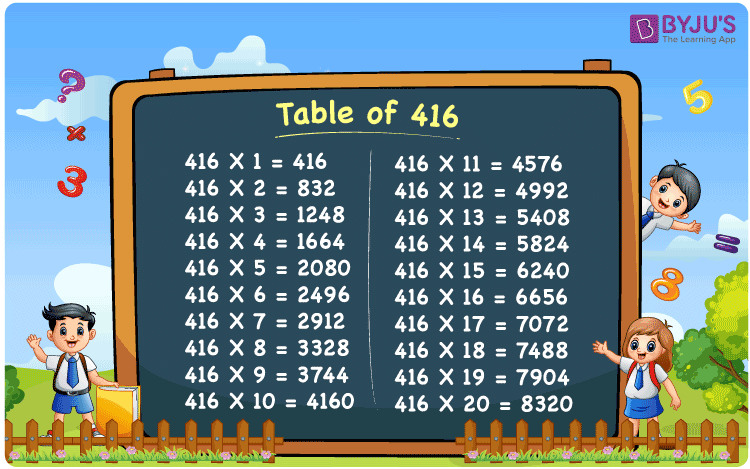Checkout JEE MAINS 2022 Question Paper Analysis : Checkout JEE MAINS 2022 Question Paper Analysis :

# Table of 416

The Multiplication Table for 416 is nothing more than the repetitive addition of the number 416. Repetitive addition is adding the same number multiple times. For example, adding 4 times 416, which is 416 + 416 + 416 + 416 is the same as 416 x 4 and that equals 1664. But adding the same number multiple times, say 8 times, can result in mistakes. Instead, the below given chart on Table for 416 can be referred to. Any multiplication table can be computed using the Multiplication Calculator.

## Table of 416 Chart## What is the 416 Times Table?

In the table of 416, we are repeatedly adding 416 multiple times. And the table below gives a repetitive addition 10 times for the number 416.

 416 × 1 = 416 416 416 × 2 = 832 416 + 416 = 832 416 × 3 = 1248 416 + 416 + 416 = 1248 416 × 4 = 1664 416 + 416 + 416 + 416 = 1664 416 × 5 = 2080 416 + 416 + 416 + 416 + 416 = 2080 416 × 6 = 2496 416 + 416 + 416 + 416 + 416 + 416 = 2496 416 × 7 = 2912 416 + 416 + 416 + 416 + 416 + 416 + 416 = 2912 416 × 8 = 3328 416 + 416 + 416 + 416 + 416 + 416 + 416 + 416 = 3328 416 × 9 = 3744 416 + 416 + 416 + 416 + 416 + 416 + 416 + 416 + 416 = 3744 416 × 10 = 4160 416 + 416 + 416 + 416 + 416 + 416 + 416 + 416 + 416 + 416 = 4160

## Multiplication Table of 416

Given below is the multiplication table of 416 being done 20 times. The below table helps you with multiplication problems that require the tables of 416.

 416 × 1 = 416 416 × 2 = 832 416 × 3 = 1248 416 × 4 = 1664 416 × 5 = 2080 416 × 6 = 2496 416 × 7 = 2912 416 × 8 = 3328 416 × 9 = 3744 416 × 10 = 4160 416 × 11 = 4576 416 × 12 = 4992 416 × 13 = 5408 416 × 14 = 5824 416 × 15 = 6240 416 × 16 = 6656 416 × 17 = 7072 416 × 18 = 7488 416 × 19 = 7904 416 × 20 = 8320

## Solved Example on Table of 416

Example:

Lohith purchased a board game which cost him Rs 416. He enjoyed the board game and wanted to give it as a gift to his friends. He has 4 friends in the group. How much money does he need so that he can give it as a gift to 4 of his friends?

Solution: Lohith needs Rs 1664 to buy 4 board games.

Cost of 1 piece of board game = 416

4 such board games = 4 x 416 = 1664.

## Frequently Asked Questions on Table of 416

### Question 1: What is 16 times 416?

Answer: 16 times 416 is 6656.

### Question 2: What are the factors of 416?

Answer: The factors of 416 are 1, 2, 4, 8, 13, 16, 26, 32, 52, 104, 208 and 416.

### Question 3: What are the prime factors of 416?

Answer: 2 and 13 are the prime factors of 416.